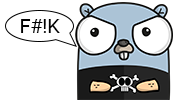#wtf go

Converting between an `interface` and a `struct` can be done leveraging type assertions in Go. Let’s take the following example code and see how it would work:

``````package main

import (
"fmt"
"math"
)

type shape interface {
area() float64
}

type rectangle struct {
length float64
width  float64
}

type circle struct {
}

func (r rectangle) area() float64 {
return r.length * r.width
}

func (c circle) area() float64 {
}

func main() {
// Create a slice of shapes to iterate over
shapes := []shape{
rectangle{length: 2, width: 2},
}

for _, item := range shapes {
if rect, ok := item.(rectangle); ok {
// Check if the item is a rectangle, if it is, then enter this if block
fmt.Println("I'm a rectangle")
fmt.Printf("length: %f, width %f, area: %f\n", rect.length, rect.width, item.area())
} else if circ, ok := item.(circle); ok {
// Check if the item is a cirlce, if it is, then enter this if block
fmt.Println("I'm a circle")
} else {
// If it's anything else, we aren't sure what it is
fmt.Println("I don't know what I am")
}
}
}
``````

When running this code you should see the following output:

``````I'm a rectangle
length: 2.000000, width 2.000000, area: 4.000000
I'm a circle